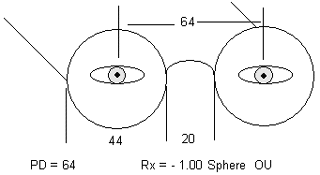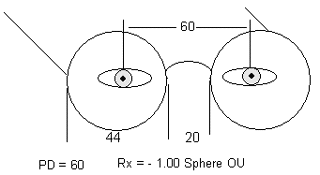< Page 5 of 17 > 1 2 3 4 5 6 7 8 9 10 11 12 13 14 15 16 17 Opthalmic Frames Horizontal Lens Decentration Example AIn this case the optical center of the lens is in the same postition as the geometric center of each eyewire. No decentration is necessary   Example BSince the GCD is 4 mm wider than the PD, the lenses must be decentered in 2 mm in each eye. This will position the MRPs to properly correspond with the pupilllary distance. The amount of decentration required in each eye is equal to the (GCD – PD) / 2 In most cases the pupillary distance of the patient will be narrower than the distance between the geometric centers of the selected frame. Less commonly, the PD will be equal to the frames geometric centers, and in rare cases the width of the PD will be greater than the GCD. In any case, to avoid unwanted prism, it is essential that the major reference points (MRPs) of the lens correspond to the pupillary distance. In the first example above, the PD and GCD are equal to 64 mm and therefore no decentration is necessary. In the second example, the GCD is 4 mm wider than the PD. By applying the formula above, it can be seen that the amount of decentration required in each lens is 2 mm. < Page 5 of 17 > 1 2 3 4 5 6 7 8 9 10 11 12 13 14 15 16 17